## Tables index

#### Thomas Mageto, John Onyango

American Journal of Applied Mathematics and Statistics. 2021, 9(2), 38-47 doi:10.12691/ajams-9-2-1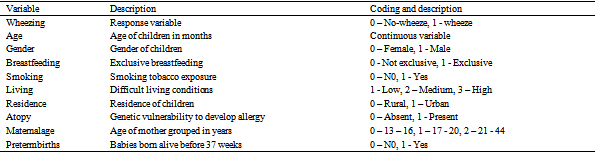• Table 1. Coding scheme of the study variables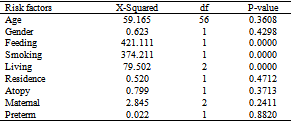• Table 2. Chi-squared test statistic and p values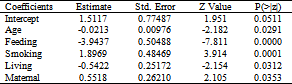• Table 3. Generalized multiple linear regression estimated parameters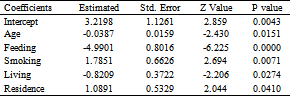• Table 4. Generalized multiple linear regression estimated parameters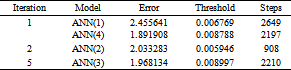• Table 5. Search for Artificial Neural Network Model in One-Hidden Layer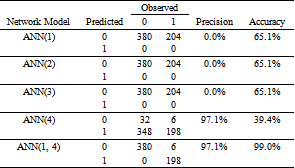• Table 6. Confusion Matrix for ANN Model with One-Hidden Layer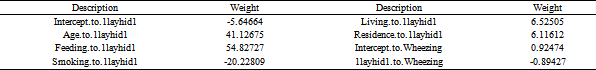• Table 7. Weights for the Artificial Neural Network Model ANN (1)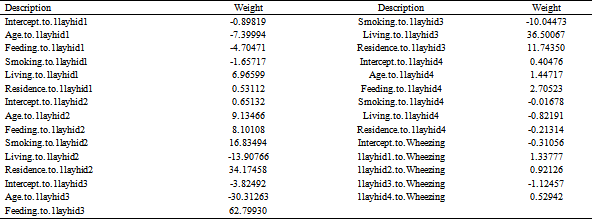• Table 8. Weights for the Artificial Neural Network Model ANN (4)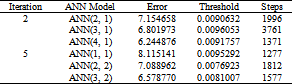• Table 9. Search for Artificial Neural Network Model in Two-Hidden Layer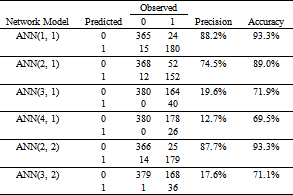• Table 10. Confusion Matrix for ANN Model with Two-Hidden Layers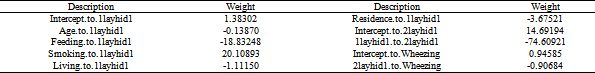• Table 11. Weights for the Artificial Neural Network Model ANN (1, 1)